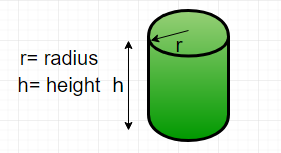# C Program for Find the perimeter of a cylinder

• Last Updated : 30 Mar, 2021

Given diameter and height, find the perimeter of a cylinder.
Perimeter is the length of the outline of a two – dimensional shape. A cylinder is a three – dimensional shape. So, technically we cannot find the perimeter of a cylinder but we can find the perimeter of the cross-section of the cylinder. This can be done by creating the projection on its base, thus, creating the projection on its side, then the shape would be reduced to a rectangle.Formula :
Perimeter of cylinder ( P ) =here d is the diameter of the cylinder
h is the height of the cylinder
Examples :

```Input : diameter = 5, height = 10
Output : Perimeter = 30

Input : diameter = 50, height = 150
Output : Perimeter = 400```

## CPP

 `// CPP program to find``// perimeter of cylinder``#include ``using` `namespace` `std;` `// Function to calculate perimeter``int` `perimeter(``int` `diameter, ``int` `height)``{``    ``return` `2 * (diameter + height);``}` `// Driver function``int` `main()``{``    ``int` `diameter = 5;``    ``int` `height = 10;``    ` `    ``cout << ``"Perimeter = "``;``    ``cout<< perimeter(diameter, height);``    ``cout<<``" units\n"``;``    ` `    ``return` `0;``}`

Output :

`Perimeter = 30 units`

Time Complexity: O(1)

Auxiliary Space: O(1)
Please refer complete article on Find the perimeter of a cylinder for more details!

My Personal Notes arrow_drop_up